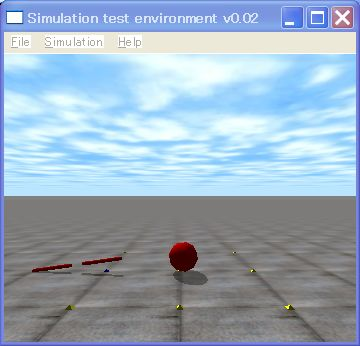# 8. ERP and CFMThis is the 8th ODE (Open Dynamics Engine) tutorial.

Using the ODE, must remember parameters, ERP (Error Reduction Parameter) and CFM (Constraint Force Mixing).

1. ERP (Error Reduction Parameter)

ERP is a parameter which corrects  joint error. As the simulation is repeated, the joint error increases due to various internal approximations.

ERP can fix the error. The value must be set between  0 and 1.  0 does not fix the error, and 1 fixes the error by the next step. Typical values are between 0.1 to 0.8, the default value is 0.2.  It is not recommended to set ERP to 1.

If you want to set the ERP, please use API as following. This API influences whole joints of the world.

• dWorldSetERP (dWorldID, dReal erp)
Set a global ERP.  The argument erp  must be set between 0.0 and 1.0.

Please insert dWorldSetERP (world, 0.0) below dWorldSetGravity() in sample7.  If ERP is set to 0,  the above robot is broken like the above figure.

2. CFM (Constraint Force Mixing)

Next,  explain CFM (Constraint Force Mixing), an another specific parameter  of the ODE, which changes stiffness of a joint.

There are two types of constraint, hard constraint and soft constraintHard constraint is the constraint which must be obeyed. Meanwhile, soft constraint is the constraint which is not so hard. Some violation can be permitted. To control the constraints, ODE uses CFM parameters. If you set CFM to 0, the hard constraint is applied, the greater value of CFM will be more soft constraint.

In addition, ODE uses a joint in the same meaning as constraint. The hard constraint means that the joint is a perfect joint. That is, the position of an anchor and the axis of a joint is not violate. In other word, the joint is hard. The soft constraint means that the joint is not perfect. In other word, the joint is flexible.

You can simulate a spring and a damper system to use ERP and CFM. The spring and the damper system are often used such as a model between a humanoid foot and the ground. Please read the ODE user manual.

Next, I explain how to use CFM. If you want to set the CFM. Please use the following API. This API, however, works all joints in the world. In the case of single-precision, the default value of the CFM is 1E-5 (1o^-5)  and in the case of precision, the value is 1E-10 (10 ^ -5).

• dWorldSetCFM (dWorldID, dReal cfm)
Set a global CFM.

Let’ use CFM.  Please insert dWorldSetCFM() below dWorldSetERP() in  the sample7.cpp. CFM changes softness of the  joint. If you want to set the value to 0,  in this case is a hard constraint. You must not set CFM to a negative value, because the system will become unstable. So please do not it.

In addition, CFM is working to stabilize the simulation. So, the system is not unstable,  increase the CFM. However, the joint will be soft. The recommended value is not greater than 1E-3 (10^-3).

That’s all.  See you next time.

スポンサーリンク
demura.netをフォローするdemura.net

### Folding@home Kanazawa (ID 257261)

みんなのためにおうちで新型コロナウイルスを解析しよう！

タイトルとURLをコピーしました